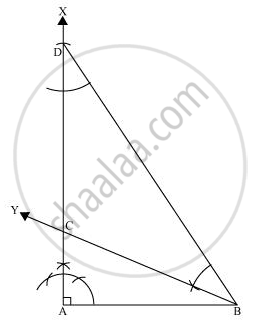# Construct a right triangle whose base is 12 cm and sum of its hypotenuse and other side is 18 cm. - Mathematics

Construct a right triangle whose base is 12 cm and sum of its hypotenuse and other side is 18 cm.

#### Solution

The below given steps will be followed to construct the required triangle.

Step I:- Draw line segment AB of 12 cm. Draw a ray AX making 90° with AB.

Step II:- Cut a line segment AD of 18 cm (as the sum of the other two sides is 18) from ray AX.

Step III:- Join DB and make an angle DBY equal to ADB.

Step IV:- Let BY intersect AX at C. Join AC, BC.

ΔABC is the required triangle.Concept: Some Constructions of Triangles
Is there an error in this question or solution?
Chapter 11: Constructions - Exercise 11.2 [Page 195]

#### APPEARS IN

NCERT Class 9 Maths
Chapter 11 Constructions
Exercise 11.2 | Q 5 | Page 195

Share